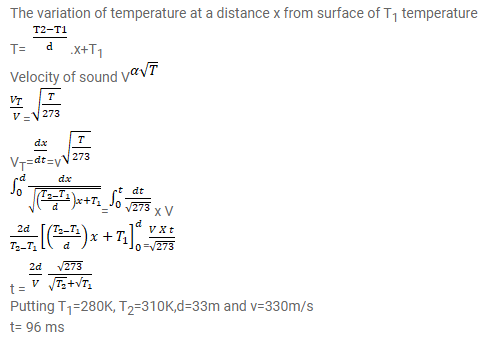# The absolute temperature of air in a region linearly increasesQuestion:

The absolute temperature of air in a region linearly increases from $T_{1}$ to $T_{2}$ in a space of width d. Find the time taken by a sound wave to go through the region in terms of $T_{1}, T_{2}, d$ and the speed $v$ of sound at $273 \mathrm{~K}$. Evaluate this time for $T_{1}=280$ $\mathrm{K}, T_{2}=310 \mathrm{~K}, d=33 \mathrm{~m}$ and $v=330 \mathrm{~m} \mathrm{~s}^{-1}$.

Solution: